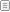<% Response.Buffer=True bd_num = Request("bd_num") act_num = Request("act_num") div_no = Request("div_no") cur_page = Request("cur_page") Set Cmd = Server.CreateObject("ADODB.Command") Cmd.ActiveConnection = ConnOle if act_num = "" then sql = "select * from BOARD_FREE where bd_num = "&bd_num&" and bd_div = "&div_no elseif act_num = "next" then sql = "select top 1 * from BOARD_FREE where bd_stat is not null and bd_num > "&bd_num&" and bd_div="&div_no&" order by bd_num asc" elseif act_num = "before" then sql = "select top 1 * from BOARD_FREE where bd_stat is not null and bd_num < "&bd_num&" and bd_div="&div_no&" order by bd_num desc" end if Cmd.CommandText = sql Cmd.CommandType = adCmdText Set rs = Cmd.Execute if not rs.eof then bd_num = rs("bd_num") div_no = rs("bd_div") bd_file = rs("bd_file") If bd_file <> "" Then bd_file = "   Ă·şÎĆÄŔĎ "&bd_file&"" End If else if act_num <> "" then response.redirect "sub07_01_view.htm?bd_num="&bd_num&"&div_no="&div_no end if response.end end if sql = "update BOARD_FREE set bd_read=bd_read+1 where bd_num = "&bd_num&" and bd_div="&div_no Cmd.CommandText = sql Cmd.CommandType = adCmdText Cmd.Execute %> <% if ev_div="" then ev_div="7" end if %>Mail From Overseas
 Free Message Board If you have any questions, or comments, we would love to hear from you use the information top of the page to contact us by e-mail Use Free Message Board as we propose otherwise We don't accepted
 <%if len(rs("bd_title")) > 120 then bd_title = Left(rs("bd_title"),120) &"..." else bd_title=rs("bd_title") end if %> <%=bd_title%> <%=rs("bd_writer")%>    Hit <%=rs("bd_read")%> <%=left(rs("bd_date"),10)%> <% if not rs("editor_ok") then response.write replace(rs("bd_content"),Chr(13),"") else response.write rs("bd_content") end if %>
 Write Modify DeletePrevious NextList